# What is 3

What is the least common denominator of 11/15 and 12/19?

Correct result:

d =  285

#### Solution: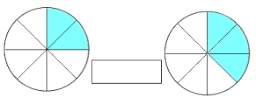We would be pleased if you find an error in the word problem, spelling mistakes, or inaccuracies and send it to us. Thank you!

Showing 1 comment:Tips to related online calculators
Do you want to calculate least common multiple two or more numbers?
Need help calculate sum, simplify or multiply fractions? Try our fraction calculator.

## Next similar math problems:

• Sum of two primesChristian Goldbach, a mathematician, found out that every even number greater than 2 can be expressed as a sum of two prime numbers. Write or express 2018 as a sum of two prime numbers.
• Simple equation 6Solve equation with one variable: X/2+X/3+X/4=X+4
• Lamps on playground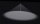The playground has the shape of a rectangle 36 x 50m. After how many meters will he place the lamps on his lighting, if the distances between them are to be the same on both sides if the builders want to use the smallest possible number of lamps?
• Plum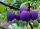On the platter are plum. How many were there if its have to be able to share equally among 8,10 and 12 children?
• Balls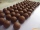From the bag with numbered balls (numbers 1,2,3,. ..20) we pick one ball. What is the probability of choosing a number containing 1?
• School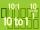Headteacher think whether the distribution of pupils in race in groups of 4,5,6,9 or 10. How many pupils must have at least school at possible options?
• Secondary school1/2 of the pupils want to study at the secondary school, 1/4 at the apprentice, 1/6 at the grammar school 3 pupils do not want to study. How many students are in the class?
• Two gears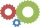Two gears with 13 and 7 teeth rotate locked into each other. How many turns does a big wheel have to make for both wheels to be in the starting position again?
• Trams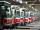Two trams started at the same time from the same place. One tram journey takes 30 minutes and the second 45 minutes to its final stop. How long will trams meet again?
• Lcm of three numbersWhat is the Lcm of 120 15 and 5
• Class 9AOn the final certificate have one quarter of the class 9A mark "C" of mathematics, seventh mark "C" from the Czech language and two students failed in chemistry. How many students attend class 9A?
• Counting numberWhat is the smallest counting number divisible by 2,5,7,8 and 15?
• Dance ensemble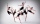The dance ensemble took the stage in pairs. During dancing, the dancers gradually formed groups of four, six and nine. How many dancers have an ensemble?
• LCM of two numberFind the smallest multiple of 63 and 147
• Tailor master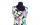There are less than 50m of textile in the tailoring workshop. When cutting on a blouse (consumption 1.5m), no textile is left. When using a cloth (consumption of 3.2m), no textile is left. How many meters of textile are in a tailor's workshop?
• The florist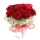A consignment of 200 roses arrived at the florist in the morning. She sold more than half of them during the day. She wants to tie bouquets of the remaining roses. If she ties a bouquet of three, four, five or six roses, there will always be one rose left
• Reminder and quotientThere are given numbers A = 135, B = 315. Find the smallest natural number R greater than 1 so that the proportions R:A, R:B are with the remainder 1.